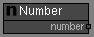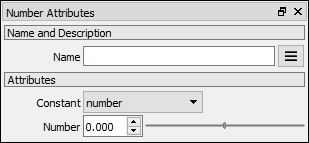# Number node

Left Toolbar >Math Nodes >Number

The Number node allows you to enter a number as an attribute and convert it to a Node Value that can flow in the node graph.

## Procedure

### To add a Number Node Value to the node graph:

1. On the left toolbar choose Math Nodes > Number.>2. Drag the Number icon into the Node Graph.3. Connect the Output: number.

No Inputs.

## Outputs

number The number value.

## Attributes PanelConstant If set to number the value returned by this node will be the number defined in the Number spinner. If set to a different constant the value returnd by this node will be the value of that constant:

pi: π

two_pi: 2.0 * π

half_pi: 0.5 * π

inv_pi: 1.0 / π

invtwopi: 1.0 / (2.0 * π)

invhalfpi: 1.0 / (0.5 * π)